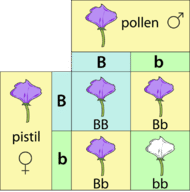# 3.15: Punnett Squares

•• Contributed by CK12
• CK12

### What's the chance of the coin landing on heads?

There is always a 50-50 chance that a coin will land on heads. Half the time it will land on heads and half the time it will land on tails. What is the chance of it landing heads twice in a row? Or three times? These rules of probability also apply to genetics.

A parent only gives one factor for each trait to an offspring. If a parent has one dominant and one recessive factor for a trait, then, on average, half the time the dominant factor will be passed on, and half the time the recessive factor will be passed on.

## Probability and Punnett Squares

A Punnett square is a special tool derived from the laws of probability. It is used to predict the possible offspring from a cross, or mating between two parents.

An example of a Punnett square (Figure below) shows the results of a cross between two purple flowers that each have one dominant factor and one recessive factor (Bb).Figure $$\PageIndex{1}$$: The Punnett square of a cross between two purple flowers (Bb). A Punnett square can be used to calculate what percentage of offspring will have a certain trait.

To create a Punnett square, perform the following steps:

1. Take the factors from the first parent and place them at the top of the square (B and b).
2. Take the factors from the second parent and line them up on the left side of the square (B and b).
3. Pull the factors from the top into the boxes below.
4. Pull the factors from the side into the boxes next to them.

The possible offspring are represented by the letters in the boxes, with one factor coming from each parent.

Results:

• Top left box: BB, or purple flowers
• Top right box: Bb, or purple flowers
• Lower left box: Bb, or purple flowers
• Lower right box: bb, or white flowers

Only one of the plants out of the four, or 25% of the plants, has white flowers (bb). The other 75% have purple flowers (BB, Bb), because the purple factor (B) is the dominant factor. This shows that the color purple is the dominant trait in pea plants.

Now imagine you cross one of the white flowers (bb) with a purple flower that has both a dominant and recessive factor (Bb). The only possible gamete in the white flower is recessive (b), while the purple flower can have gametes with either dominant (B) or recessive (b).

Practice using a Punnett square with this cross (see Table below).

b b
B Bb Bb
b bb bb

Did you find that 50% of the offspring will be purple, and 50% of the offspring will be white?

## Summary

• A Punnett square is a special tool used to predict the offspring from a cross, or mating between two parents.
• In a Punnett square, the possible offspring are represented by the letters in the boxes, with one factor coming from each parent.

## Explore More

Use the resources below to answer the questions that follow.

### Explore More I

1. What are Mendel's two rules of heredity?
2. If a pure bred black rabbit and a white rabbit mate, what will their potential offspring look like?
3. If two hybrid Ff rabbits mate, what will the offspring look like?

### Explore More II

1. If you cross an Aa individual with another Aa individual, what will the genotype ratio be in the next generation? What will be the phenotype ratio?
2. If you cross an AABb individual with an Aabb individual, what will the genotype ratio be in the next generation? What will be the phenotype ratio?
3. If you cross an AAbb individual with an aabb individual, what will the genotype ratio be in the next generation? What will be the phenotype ratio?

Review

1. In peas, yellow seeds (Y) are dominant over green seeds (y). If a yy plant is crossed with a YY plant, what ratio of plants in the offspring would you predict?
2. What ratio of plants in the offspring would you predict from a Yy x Yy cross?
3. In guinea pigs, smooth coat (S) is dominant over rough coat (s). If an SS guinea pig is crossed with an ss guinea pig, what ratio of guinea pigs in the offspring would you predict?
4. What ratio of guinea pigs in the offspring would you predict from a Ss x ss cross?